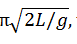Click to Chat

1800-1023-196

+91-120-4616500

CART 0

• 0

MY CART (5)

Use Coupon: CART20 and get 20% off on all online Study Material

ITEM
DETAILS
MRP
DISCOUNT
FINAL PRICE
Total Price: Rs.

There are no items in this cart.
Continue Shopping
`        A U-tube is filled with a single homogeneous liquid. The liquid is temporarily depressed in one side by a piston. The piston is removed and the level of liquid in each side oscillates. Show that the period of oscillation is π, where L is the total length of the liquid in the tube.`
4 years ago

```							In an oscillating system, the restoring force F is defined as,F = kxHere k is the force constant and x is the displacement of the particle from its mean position.The restoring force F acting on the liquid is defined as,F = 2ρAxgHere ρ is the density of the liquid, A is the area of cross section, x is the height from equilibrium position and g is the acceleration of free fall.If we compare the equation F = kx with F = 2ρAxg, the force constant k will be,k = 2ρAgDensity (ρ) is equal to mass (m) per unit volume (V).ρ = m/ VSo using equation ρ = m/ V, mass m of the fluid in the U-tube having area A and total length of the liquid L will be,ρ = m/ Vm= ρ V = ρ(AL) (Since V= AL)To find out the period of oscillation T, substitute ρ(AL) for m and 2ρAg for k in the equation T = 2π √m/k,T = 2π √m/k = 2π √( ρ(AL) /(2ρAg) = π√2L/gFrom the above observation we conclude that, period of oscillation will be π√2L/g.
```
4 years ago
Think You Can Provide A Better Answer ?

Other Related Questions on Wave Motion

View all Questions »Course Features

• 101 Video Lectures
• Revision Notes
• Previous Year Papers
• Mind Map
• Study Planner
• NCERT Solutions
• Discussion Forum
• Test paper with Video SolutionCourse Features

• 110 Video Lectures
• Revision Notes
• Test paper with Video Solution
• Mind Map
• Study Planner
• NCERT Solutions
• Discussion Forum
• Previous Year Exam Questions